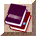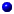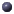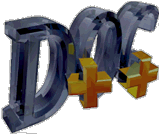##class <Samples> Samples : public TclObject

a set of statistical samples

## Inheritance:

### Public Methodsint cnt()int command(int argc, const char*const* argv)double mean()void newPoint(double val)void reset()Samples()double sum()double variance()

### Protected Fieldsint cnt_
count of samplesdouble sqsum_
sum of (x_i)^2double sum_
sum of x_i

## Documentation

a set of statistical samplesSamples()void newPoint(double val)int cnt()double sum()double mean()double variance()void reset()int command(int argc, const char*const* argv)int cnt_
count of samplesdouble sum_
sum of x_idouble sqsum_
sum of (x_i)^2

This class has no child classes.